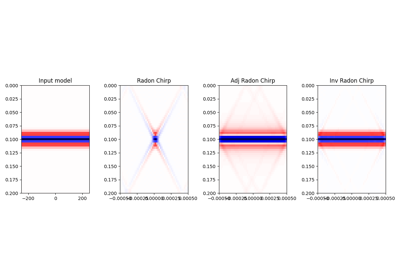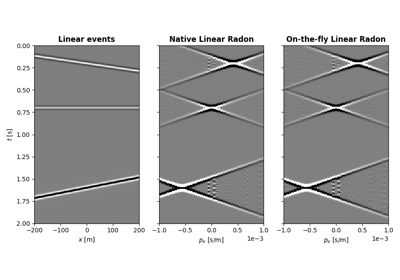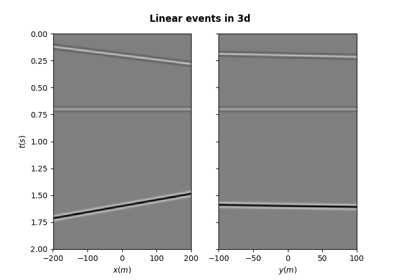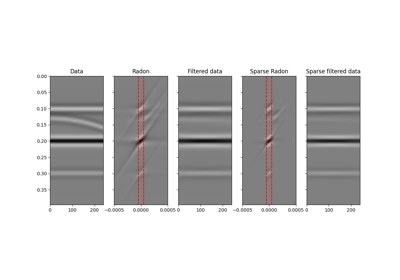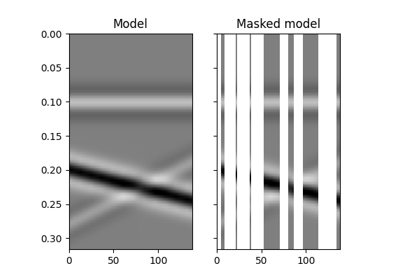# pylops.utils.seismicevents.linear2d¶

pylops.utils.seismicevents.linear2d(x, t, v, t0, theta, amp, wav)[source]

Linear 2D events

Create 2d linear events given propagation velocity, intercept time, angle, and amplitude of each event

Parameters: x : numpy.ndarray space axis t : numpy.ndarray time axis v : float propagation velocity t0 : intercept time at $$x=0$$ of each linear event theta : angle (in degrees) of each linear event amp : amplitude of each linear event wav : numpy.ndarray wavelet to be applied to data d : numpy.ndarray data without wavelet of size $$[n_x \times n_t]$$ dwav : numpy.ndarray data with wavelet of size $$[n_x \times n_t]$$

Notes

Each event is created using the following relation:

$t_i(x) = t_{0,i} + p_{x,i} x$

where $$p_{x,i}=\sin( \theta_i)/v$$

## Examples using pylops.utils.seismicevents.linear2d¶